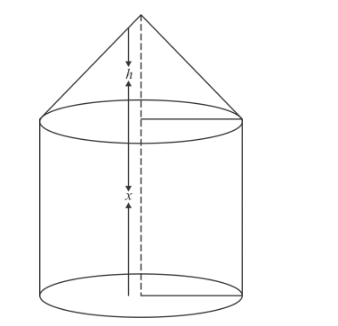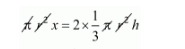# A solid consists of a circular cylinder with an exact fitting right circular cone placed at the top.`
Question:

A solid consists of a circular cylinder with an exact fitting right circular cone placed at the top. The height of the cone is h. If the total volume of the solid is 3 times the volume of the cone, then the height of the circular is

(a) $2 h$

(b) $\frac{2 h}{3}$

(c) $\frac{3 h}{2}$

(d) $4 h$

Solution:Let r be the radius of the base of solid.

Clearly,

The volume of solid = 3 × volume of cone

Vol. of cone + Vol. of cylinder = 3 Volume of cone

Vol. of cylinder = 2 Vol. of cone$x=\frac{2}{3} h$

Thus,

The height of cylinder $=\frac{2 h}{3}$

Hence, the correct answer is choice (b).